﻿ How do I calculate the volume of my pool so I know how much activator D to use?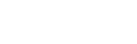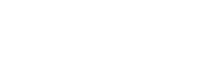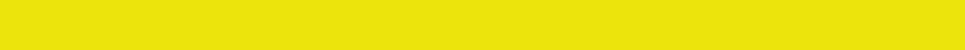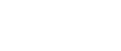Website developed by VirtualWebsolutions.co.za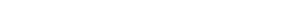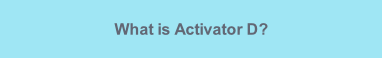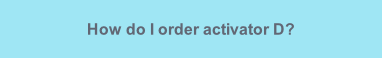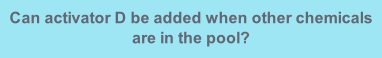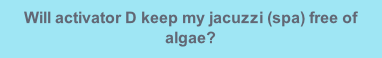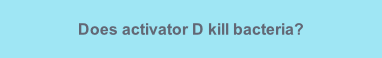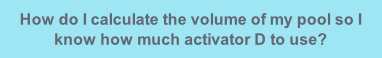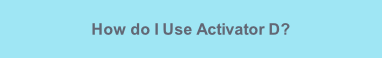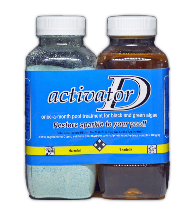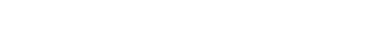How do I calculate the volume of my pool so I know how much activator D to use?

A useful rule-of-thumb is that most pools will have a volume of roughly 1.5 x their area. This usually accounts for the average of the deep and shallow ends.

Example

1. Pace out the length and breadth of your pool   L=8m  B=4m

2. Multiply the 2 numbers together to get the area  8 x 4 = 32

3. Multiply the area by 1.5  32 x 1.5 = 48

4. Multiply the answer by 1000 to get litres  48 000 litres

A pool of roughly 50 000 litres will require one treatment of activator D each month.

If your pool is approximately 25 000 litres (between 20 000 - 30 000) you will only need to add half of each bottle of the activator D duo to your pool each month to keep it sparkling clean and algae free.